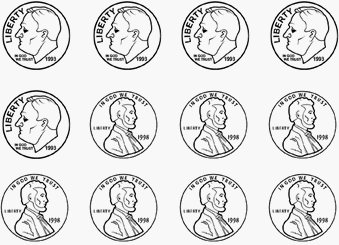Skill 8E
Using the Fewest Number of Coins to equal an Amount

When paying for an item (or making change) in a real life situation, the typical procedure is to use the fewest number of coins.  The problem assesses this skill.

Count the value of coins below and write it down on a piece of paper.
After you do this answer the question below the coins.What is the the fewest number of coins you can use
to equal the same amount?
 ________ ________ ________ ________ quarters dimes nickels pennies